### Learning Objectives

• Calculate the limit of a function as $x$ increases or decreases without bound.
• Recognize a horizontal asymptote on the graph of a function.
• Estimate the end behavior of a function as $x$ increases or decreases without bound.
• Recognize an oblique asymptote on the graph of a function.

To graph a function $f$ defined on an unbounded domain, we also need to know the behavior of $f$ as $x\to \pm \infty .$ In this section, we define limits at infinity and show how these limits affect the graph of a function.

# Limits at Infinity

We begin by examining what it means for a function to have a finite limit at infinity. Then we study the idea of a function with an infinite limit at infinity . Back in Introduction to Functions and Graphs , we looked at vertical asymptotes; in this section we deal with horizontal and oblique asymptotes.

## Limits at Infinity and Horizontal Asymptotes

Recall that $\underset{x\to a}{\text{lim}}f(x)=L$ means $f(x)$ becomes arbitrarily close to $L$ as long as $x$ is sufficiently close to $a.$ We can extend this idea to limits at infinity. For example, consider the function $f(x)=2+\frac{1}{x}.$ As can be seen graphically in (Figure) and numerically in (Figure) , as the values of $x$ get larger, the values of $f(x)$ approach 2. We say the limit as $x$ approaches $\infty$ of $f(x)$ is 2 and write $\underset{x\to \infty }{\text{lim}}f(x)=2.$ Similarly, for $x < 0,$ as the values $|x|$ get larger, the values of $f(x)$ approaches 2. We say the limit as $x$ approaches $-\infty$ of $f(x)$ is 2 and write $\underset{x\to a}{\text{lim}}f(x)=2.$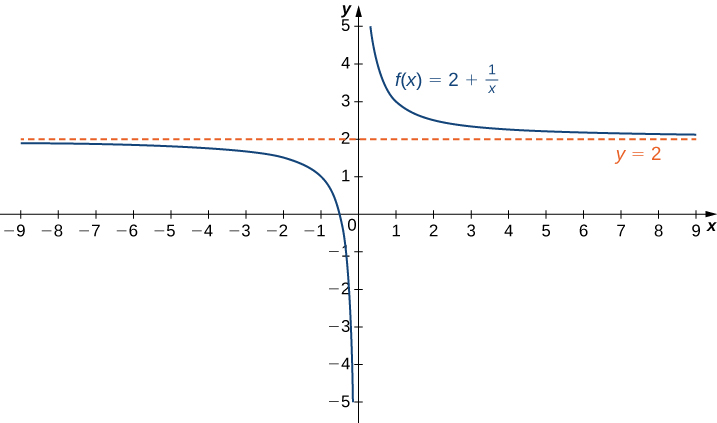Figure 1. The function approaches the asymptote $y=2$ as $x$ approaches $\pm \infty .$

 $x$ 10 100 1,000 10,000 $2+\frac{1}{x}$ 2.1 2.01 2.001 2.0001 $x$ -10 -100 -1000 -10,000 $2+\frac{1}{x}$ 1.9 1.99 1.999 1.9999

More generally, for any function $f,$ we say the limit as $x\to \infty$ of $f(x)$ is $L$ if $f(x)$ becomes arbitrarily close to $L$ as long as $x$ is sufficiently large. In that case, we write $\underset{x\to a}{\text{lim}}f(x)=L.$ Similarly, we say the limit as $x\to -\infty$ of $f(x)$ is $L$ if $f(x)$ becomes arbitrarily close to $L$ as long as $x < 0$ and $|x|$ is sufficiently large. In that case, we write $\underset{x\to -\infty }{\text{lim}}f(x)=L.$ We now look at the definition of a function having a limit at infinity.

### Definition

(Informal) If the values of $f(x)$ become arbitrarily close to $L$ as $x$ becomes sufficiently large, we say the function $f$ has a limit at infinity and write

$\underset{x\to \infty }{\text{lim}}f(x)=L.$

If the values of $f(x)$ becomes arbitrarily close to $L$ for $x < 0$ as $|x|$ becomes sufficiently large, we say that the function $f$ has a limit at negative infinity and write

$\underset{x\to \infty }{\text{lim}}f(x)=L.$

If the values $f(x)$ are getting arbitrarily close to some finite value $L$ as $x\to \infty$ or $x\to -\infty ,$ the graph of $f$ approaches the line $y=L.$ In that case, the line $y=L$ is a horizontal asymptote of $f$ ( (Figure) ). For example, for the function $f(x)=\frac{1}{x},$ since $\underset{x\to \infty }{\text{lim}}f(x)=0,$ the line $y=0$ is a horizontal asymptote of $f(x)=\frac{1}{x}.$

### Definition

If $\underset{x\to \infty }{\text{lim}}f(x)=L$ or $\underset{x\to -\infty }{\text{lim}}f(x)=L,$ we say the line $y=L$ is a horizontal asymptote of $f.$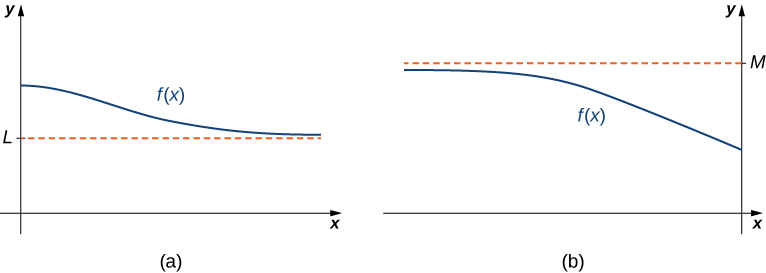Figure 2. (a) As $x\to \infty ,$ the values of $f$ are getting arbitrarily close to $L.$ The line $y=L$ is a horizontal asymptote of $f.$ (b) As $x\to -\infty ,$ the values of $f$ are getting arbitrarily close to $M.$ The line $y=M$ is a horizontal asymptote of $f.$

A function cannot cross a vertical asymptote because the graph must approach infinity (or $-\infty )$ from at least one direction as $x$ approaches the vertical asymptote. However, a function may cross a horizontal asymptote. In fact, a function may cross a horizontal asymptote an unlimited number of times. For example, the function $f(x)=\frac{( \cos x)}{x}+1$ shown in (Figure) intersects the horizontal asymptote $y=1$ an infinite number of times as it oscillates around the asymptote with ever-decreasing amplitude.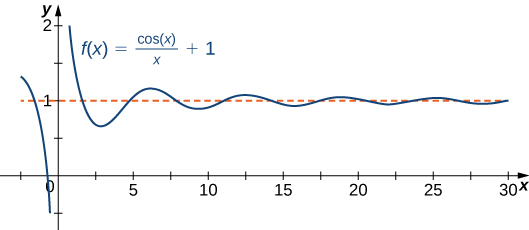Figure 3. The graph of $f(x)=( \cos x)\text{/}x+1$ crosses its horizontal asymptote $y=1$ an infinite number of times.

The algebraic limit laws and squeeze theorem we introduced in Introduction to Limits also apply to limits at infinity. We illustrate how to use these laws to compute several limits at infinity.

### Computing Limits at Infinity

For each of the following functions $f,$ evaluate $\underset{x\to \infty }{\text{lim}}f(x)$ and $\underset{x\to -\infty }{\text{lim}}f(x).$ Determine the horizontal asymptote(s) for $f.$

1. $f(x)=5-\frac{2}{{x}^{2}}$
2. $f(x)=\frac{ \sin x}{x}$
3. $f(x)={ \tan }^{-1}(x)$

#### Solution

1. Using the algebraic limit laws, we have $\underset{x\to \infty }{\text{lim}}(5-\frac{2}{{x}^{2}})=\underset{x\to \infty }{\text{lim}}5-2(\underset{x\to \infty }{\text{lim}}\frac{1}{x}).(\underset{x\to \infty }{\text{lim}}\frac{1}{x})=5-2\cdot 0=5.$Similarly, $\underset{x\to \infty }{\text{lim}}f(x)=5.$Therefore, $f(x)=5-\frac{2}{{x}^{2}}$ has a horizontal asymptote of $y=5$ and $f$ approaches this horizontal asymptote as $x\to \pm \infty$ as shown in the following graph.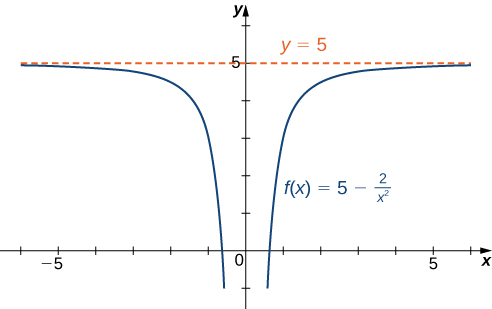Figure 4. This function approaches a horizontal asymptote as $x\to \pm \infty .$

2. Since $-1\le \sin x\le 1$ for all $x,$ we have
$\frac{-1}{x}\le \frac{ \sin x}{x}\le \frac{1}{x}$

for all $x\ne 0.$ Also, since

$\underset{x\to \infty }{\text{lim}}\frac{-1}{x}=0=\underset{x\to \infty }{\text{lim}}\frac{1}{x},$

we can apply the squeeze theorem to conclude that

$\underset{x\to \infty }{\text{lim}}\frac{ \sin x}{x}=0.$

Similarly,

$\underset{x\to -\infty }{\text{lim}}\frac{ \sin x}{x}=0.$

Thus, $f(x)=\frac{ \sin x}{x}$ has a horizontal asymptote of $y=0$ and $f(x)$ approaches this horizontal asymptote as $x\to \pm \infty$ as shown in the following graph.

3. To evaluate $\underset{x\to \infty }{\text{lim}}{ \tan }^{-1}(x)$ and $\underset{x\to -\infty }{\text{lim}}{ \tan }^{-1}(x),$ we first consider the graph of $y= \tan (x)$ over the interval $(-\pi \text{/}2,\pi \text{/}2)$ as shown in the following graph.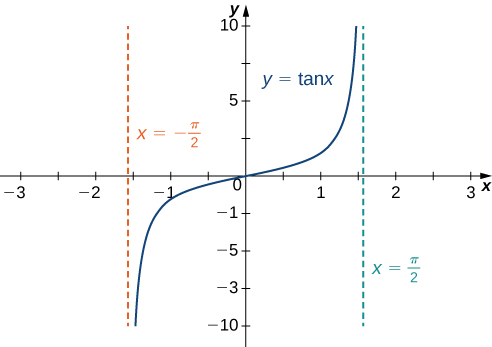The graph of $\tan x$ has vertical asymptotes at $x=\pm \frac{\pi }{2}$

Since

$\underset{x\to {(\pi \text{/}2)}^{-}}{\text{lim}} \tan x=\infty ,$

it follows that

$\underset{x\to \infty }{\text{lim}}{ \tan }^{-1}(x)=\frac{\pi }{2}.$

Similarly, since

$\underset{x\to {(\pi \text{/}2)}^{+}}{\text{lim}} \tan x=-\infty ,$

it follows that

$\underset{x\to -\infty }{\text{lim}}{ \tan }^{-1}(x)=-\frac{\pi }{2}.$

As a result, $y=\frac{\pi }{2}$ and $y=-\frac{\pi }{2}$ are horizontal asymptotes of $f(x)={ \tan }^{-1}(x)$ as shown in the following graph.

Evaluate $\underset{x\to -\infty }{\text{lim}}(3+\frac{4}{x})$ and $\underset{x\to \infty }{\text{lim}}(3+\frac{4}{x}).$ Determine the horizontal asymptotes of $f(x)=3+\frac{4}{x},$ if any.

#### Hint

$\underset{x\to \pm \infty }{\text{lim}}1\text{/}x=0$

#### Solution

Both limits are 3. The line $y=3$ is a horizontal asymptote.

## Infinite Limits at Infinity

Sometimes the values of a function $f$ become arbitrarily large as $x\to \infty$ (or as $x\to -\infty ).$ In this case, we write $\underset{x\to \infty }{\text{lim}}f(x)=\infty$ (or $\underset{x\to -\infty }{\text{lim}}f(x)=\infty ).$ On the other hand, if the values of $f$ are negative but become arbitrarily large in magnitude as $x\to \infty$ (or as $x\to -\infty ),$ we write $\underset{x\to \infty }{\text{lim}}f(x)=-\infty$ (or $\underset{x\to -\infty }{\text{lim}}f(x)=-\infty ).$

For example, consider the function $f(x)={x}^{3}.$ As seen in (Figure) and (Figure) , as $x\to \infty$ the values $f(x)$ become arbitrarily large. Therefore, $\underset{x\to \infty }{\text{lim}}{x}^{3}=\infty .$ On the other hand, as $x\to -\infty ,$ the values of $f(x)={x}^{3}$ are negative but become arbitrarily large in magnitude. Consequently, $\underset{x\to -\infty }{\text{lim}}{x}^{3}=-\infty .$

 $x$ 10 20 50 100 1000 ${x}^{3}$ 1000 8000 125,000 1,000,000 1,000,000,000 $x$ -10 -20 -50 -100 -1000 ${x}^{3}$ -1000 -8000 -125,000 -1,000,000 -1,000,000,000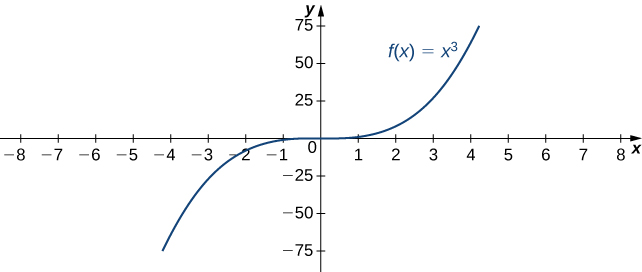Figure 8. For this function, the functional values approach infinity as $x\to \pm \infty .$

### Definition

(Informal) We say a function $f$ has an infinite limit at infinity and write

$\underset{x\to \infty }{\text{lim}}f(x)=\infty .$

if $f(x)$ becomes arbitrarily large for $x$ sufficiently large. We say a function has a negative infinite limit at infinity and write

$\underset{x\to \infty }{\text{lim}}f(x)=-\infty .$

if $f(x) < 0$ and $|f(x)|$ becomes arbitrarily large for $x$ sufficiently large. Similarly, we can define infinite limits as $x\to -\infty .$

## Formal Definitions

Earlier, we used the terms arbitrarily close , arbitrarily large , and sufficiently large to define limits at infinity informally. Although these terms provide accurate descriptions of limits at infinity, they are not precise mathematically. Here are more formal definitions of limits at infinity. We then look at how to use these definitions to prove results involving limits at infinity.

### Definition

(Formal) We say a function $f$ has a limit at infinity, if there exists a real number $L$ such that for all $\epsilon < 0,$ there exists $N < 0$ such that

$|f(x)-L| < \epsilon$

for all $x < N.$ In that case, we write

$\underset{x\to \infty }{\text{lim}}f(x)=L$

(see (Figure) ).

We say a function $f$ has a limit at negative infinity if there exists a real number $L$ such that for all $\epsilon < 0,$ there exists $N < 0$ such that

$|f(x)-L| < \epsilon$

for all $x < N.$ In that case, we write

$\underset{x\to -\infty }{\text{lim}}f(x)=L.$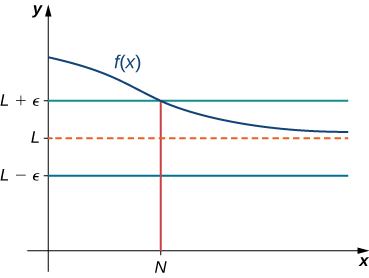Figure 9. For a function with a limit at infinity, for all $x < N,$ $|f(x)-L| < \epsilon .$

Earlier in this section, we used graphical evidence in (Figure) and numerical evidence in (Figure) to conclude that $\underset{x\to \infty }{\text{lim}}(\frac{2+1}{x})=2.$ Here we use the formal definition of limit at infinity to prove this result rigorously.

### A Finite Limit at Infinity Example

Use the formal definition of limit at infinity to prove that $\underset{x\to \infty }{\text{lim}}(\frac{2+1}{x})=2.$

#### Solution

Let $\epsilon < 0.$ Let $N=\frac{1}{\epsilon }.$ Therefore, for all $x < N,$ we have

$|2+\frac{1}{x}-2|=|\frac{1}{x}|=\frac{1}{x} < \frac{1}{N}=\epsilon \text{.}$

Use the formal definition of limit at infinity to prove that $\underset{x\to \infty }{\text{lim}}(\frac{3-1}{{x}^{2}})=3.$

#### Hint

Let $N=\frac{1}{\sqrt{\epsilon }}.$

#### Solution

Let $\epsilon < 0.$ Let $N=\frac{1}{\sqrt{\epsilon }}.$ Therefore, for all $x < N,$ we have

$|3-\frac{1}{{x}^{2}}-3|=\frac{1}{{x}^{2}} < \frac{1}{{N}^{2}}=\epsilon$

Therefore, $\underset{x\to \infty }{\text{lim}}(3-1\text{/}{x}^{2})=3.$

We now turn our attention to a more precise definition for an infinite limit at infinity.

### Definition

(Formal) We say a function $f$ has an infinite limit at infinity and write

$\underset{x\to \infty }{\text{lim}}f(x)=\infty$

if for all $M < 0,$ there exists an $N < 0$ such that

$f(x) < M$

for all $x < N$ (see (Figure) ).

We say a function has a negative infinite limit at infinity and write

$\underset{x\to \infty }{\text{lim}}f(x)=-\infty$

if for all $M < 0,$ there exists an $N < 0$ such that

$f(x) < M$

for all $x < N.$

Similarly we can define limits as $x\to -\infty .$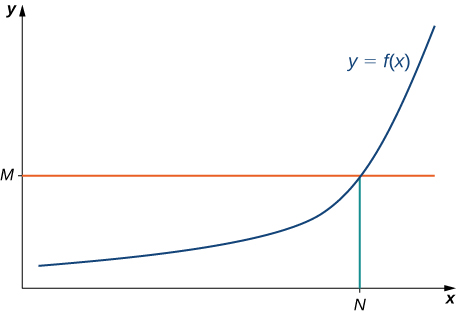Figure 10. For a function with an infinite limit at infinity, for all $x < N,$ $f(x) < M.$

Earlier, we used graphical evidence ( (Figure) ) and numerical evidence ( (Figure) ) to conclude that $\underset{x\to \infty }{\text{lim}}{x}^{3}=\infty .$ Here we use the formal definition of infinite limit at infinity to prove that result.

### An Infinite Limit at Infinity

Use the formal definition of infinite limit at infinity to prove that $\underset{x\to \infty }{\text{lim}}{x}^{3}=\infty .$

#### Solution

Let $M < 0.$ Let $N=\sqrt{M}.$ Then, for all $x < N,$ we have

${x}^{3} < {N}^{3}={(\sqrt{M})}^{3}=M.$

Therefore, $\underset{x\to \infty }{\text{lim}}{x}^{3}=\infty .$

Use the formal definition of infinite limit at infinity to prove that $\underset{x\to \infty }{\text{lim}}3{x}^{2}=\infty .$

#### Hint

Let $N=\sqrt{\frac{M}{3}}.$

#### Solution

Let $M < 0.$ Let $N=\sqrt{\frac{M}{3}}.$ Then, for all $x < N,$ we have

$3{x}^{2} < 3{N}^{2}=3{(\sqrt{\frac{M}{3}})}^{2}{2}^{}=\frac{3M}{3}=M$

# End Behavior

The behavior of a function as $x\to \pm\infty$ is called the function’s end behavior . At each of the function’s ends, the function could exhibit one of the following types of behavior:

1. The function $f(x)$ approaches a horizontal asymptote $y=L.$
2. The function $f(x)\to \infty$ or $f(x)\to -\infty .$
3. The function does not approach a finite limit, nor does it approach $\infty$ or $-\infty .$ In this case, the function may have some oscillatory behavior.

Let’s consider several classes of functions here and look at the different types of end behaviors for these functions.

## End Behavior for Polynomial Functions

Consider the power function $f(x)={x}^{n}$ where $n$ is a positive integer. From (Figure) and (Figure) , we see that

$\underset{x\to \infty }{\text{lim}}{x}^{n}=\infty ;n=1,2,3\text{,…}$

and

$\underset{x\to -\infty }{\text{lim}}{x}^{n}=\bigg\{\begin{array}{c}\infty ;n=2,4,6\text{,…}\hfill \\ -\infty ;n=1,3,5\text{,…}\hfill \end{array}.$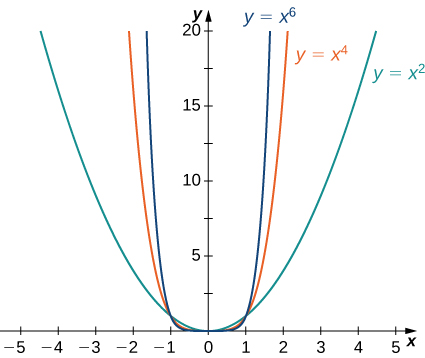Figure 11. For power functions with an even power of $n,$ $\underset{x\to \infty }{\text{lim}}{x}^{n}=\infty =\underset{x\to -\infty }{\text{lim}}{x}^{n}.$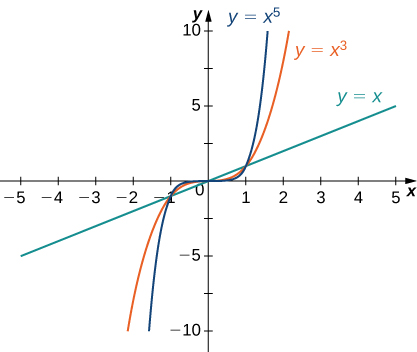Figure 12. For power functions with an odd power of $n,$ $\underset{x\to \infty }{\text{lim}}{x}^{n}=\infty$ and $\underset{x\to -\infty }{\text{lim}}{x}^{n}=-\infty .$

Using these facts, it is not difficult to evaluate $\underset{x\to \infty }{\text{lim}}c{x}^{n}$ and $\underset{x\to -\infty }{\text{lim}}c{x}^{n},$ where $c$ is any constant and $n$ is a positive integer. If $c < 0,$ the graph of $y=c{x}^{n}$ is a vertical stretch or compression of $y={x}^{n},$ and therefore

$\underset{x\to \infty }{\text{lim}}c{x}^{n}=\underset{x\to \infty }{\text{lim}}{x}^{n}\text{ and }\underset{x\to -\infty }{\text{lim}}c{x}^{n}=\underset{x\to -\infty }{\text{lim}}{x}^{n}\text{ if }c < 0.$

If $c < 0,$ the graph of $y=c{x}^{n}$ is a vertical stretch or compression combined with a reflection about the $x$-axis, and therefore

$\underset{x\to \infty }{\text{lim}}c{x}^{n}=-\underset{x\to \infty }{\text{lim}}{x}^{n}\text{ and }\underset{x\to -\infty }{\text{lim}}c{x}^{n}=-\underset{x\to -\infty }{\text{lim}}{x}^{n}\text{ if }c < 0.$

If $c=0,y=c{x}^{n}=0,$ in which case $\underset{x\to \infty }{\text{lim}}c{x}^{n}=0=\underset{x\to -\infty }{\text{lim}}c{x}^{n}.$

### Limits at Infinity for Power Functions

For each function $f,$ evaluate $\underset{x\to \infty }{\text{lim}}f(x)$ and $\underset{x\to -\infty }{\text{lim}}f(x).$

1. $f(x)=-5{x}^{3}$
2. $f(x)=2{x}^{4}$
3. $f(x)=2{x}^{3}-{x}^{2}$

#### Solution

1. Since the coefficient of ${x}^{3}$ is -5, the graph of $f(x)=-5{x}^{3}$ involves a vertical stretch and reflection of the graph of $y={x}^{3}$ about the $x$-axis. Therefore, $\underset{x\to \infty }{\text{lim}}(-5{x}^{3})=-\infty$ and $\underset{x\to -\infty }{\text{lim}}(-5{x}^{3})=\infty .$
2. Since the coefficient of ${x}^{4}$ is 2, the graph of $f(x)=2{x}^{4}$ is a vertical stretch of the graph of $y={x}^{4}.$ Therefore, $\underset{x\to \infty }{\text{lim}}2{x}^{4}=\infty$ and $\underset{x\to -\infty }{\text{lim}}2{x}^{4}=\infty .$
3. This is an $\infty - \infty$ form so limit laws don’t help. However, by factoring we get
$\underset{x\to \pm\infty }{\text{lim}}\left( 2x^3-x^2 \right) = \underset{x\to \pm\infty }{\text{lim}}x^3\left( 2-\frac{1}{x} \right).$As $x\to\infty$, the power $x^3 \to \infty$ where the second factor gets close to $2$. Hence the limit is $\infty.$As $x\to -\infty$, the power $x^3 \to -\infty$ where the second factor gets close to $2$. Hence the limit is $-\infty.$

Let $f(x)=-3{x}^{4}+4x^3.$ Find $\underset{x\to \infty }{\text{lim}}f(x).$

#### Hint

The coefficient -3 is negative.

#### Solution

$-\infty$

## End Behavior for Algebraic Functions

The end behavior for rational functions and functions involving radicals is a little more complicated than for polynomials. To evaluate the limits at infinity for a rational function, we divide the numerator and denominator by the highest power of $x$ appearing in the denominator. This determines which term in the overall expression dominates the behavior of the function at large values of $x.$

### Determining End Behavior for Rational Functions

For each of the following functions, determine the limits as $x\to \infty$ and $x\to -\infty .$ Then, use this information to describe the end behavior of the function.

1. $f(x)=\frac{3x-1}{2x+5}$
2. $f(x)=\frac{3{x}^{2}+2x}{4{x}^{3}-5x+7}$
3. $f(x)=\frac{3{x}^{2}+4x}{x+2}$

#### Solution

1. The highest power of $x$ in the denominator is $x.$ Therefore, dividing the numerator and denominator by $x$ and applying the algebraic limit laws, we see that
$\begin{array}{cc}\hfill \underset{x\to \pm \infty }{\text{lim}}\frac{3x-1}{2x+5}& =\underset{x\to \pm \infty }{\text{lim}}\frac{3-1\text{/}x}{2+5\text{/}x}\hfill \\ & =\frac{\underset{x\to \pm \infty }{\text{lim}}(3-1\text{/}x)}{\underset{x\to \pm \infty }{\text{lim}}(2+5\text{/}x)}\hfill \\ & =\frac{\underset{x\to \pm \infty }{\text{lim}}3-\underset{x\to \pm \infty }{\text{lim}}1\text{/}x}{\underset{x\to \pm \infty }{\text{lim}}2+\underset{x\to \pm \infty }{\text{lim}}5\text{/}x}\hfill \\ & =\frac{3-0}{2+0}=\frac{3}{2}.\hfill \end{array}$

Since $\underset{x\to \pm \infty }{\text{lim}}f(x)=\frac{3}{2},$ we know that $y=\frac{3}{2}$ is a horizontal asymptote for this function as shown in the following graph.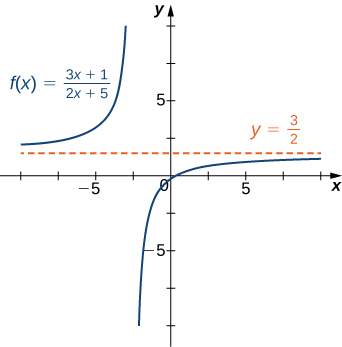Figure 14. The graph of this rational function approaches a horizontal asymptote as $x\to \pm \infty .$

2. Since the largest power of $x$ appearing in the denominator is ${x}^{3},$ divide the numerator and denominator by ${x}^{3}.$ After doing so and applying algebraic limit laws, we obtain
$\underset{x\to \pm \infty }{\text{lim}}\frac{3{x}^{2}+2x}{4{x}^{3}-5x+7}=\underset{x\to \pm \infty }{\text{lim}}\frac{3\text{/}x+2\text{/}{x}^{2}}{4-5\text{/}{x}^{2}+7\text{/}{x}^{3}}=\frac{3.0+2.0}{4-5.0+7.0}=0.$

Therefore $f$ has a horizontal asymptote of $y=0$ as shown in the following graph.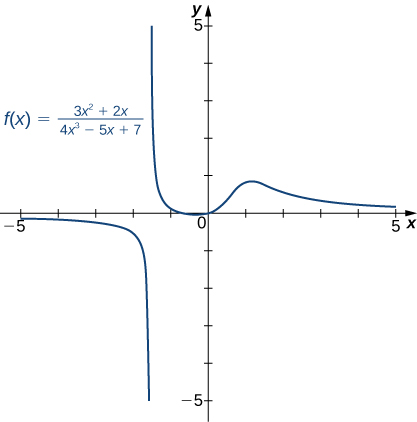Figure 15. The graph of this rational function approaches the horizontal asymptote $y=0$ as $x\to \pm \infty .$

3. Dividing the numerator and denominator by $x,$ we have
$\underset{x\to \pm \infty }{\text{lim}}\frac{3{x}^{2}+4x}{x+2}=\underset{x\to \pm \infty }{\text{lim}}\frac{3x+4}{1+2\text{/}x}.$

As $x\to \pm \infty ,$ the denominator approaches 1. As $x\to \infty ,$ the numerator approaches $+\infty .$ As $x\to -\infty ,$ the numerator approaches $-\infty .$ Therefore $\underset{x\to \infty }{\text{lim}}f(x)=\infty ,$ whereas $\underset{x\to -\infty }{\text{lim}}f(x)=-\infty$ as shown in the following figure.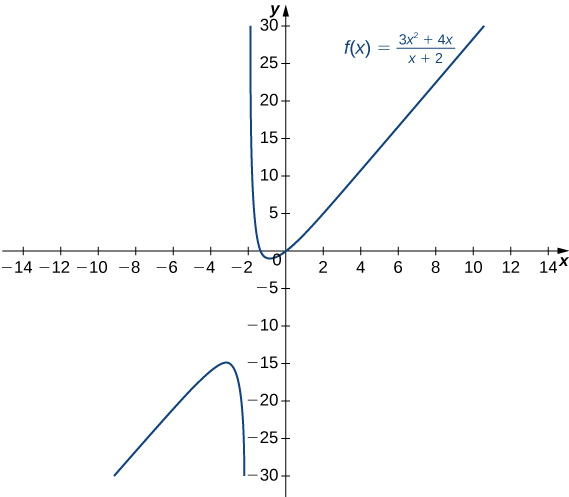Figure 16. As $x\to \infty ,$ the values $f(x)\to \infty .$ As $x\to -\infty ,$ the values $f(x)\to -\infty .$

Evaluate $\underset{x\to \pm \infty }{\text{lim}}\frac{3{x}^{2}+2x-1}{5{x}^{2}-4x+7}$ and use these limits to determine the end behavior of $f(x)=\frac{3{x}^{2}+2x-2}{5{x}^{2}-4x+7}.$

#### Hint

Divide the numerator and denominator by ${x}^{2}.$

#### Solution

$\frac{3}{5}$

Before proceeding, consider the graph of $f(x)=\frac{(3{x}^{2}+4x)}{(x+2)}$ shown in (Figure) . As $x\to \infty$ and $x\to -\infty ,$ the graph of $f$ appears almost linear. Although $f$ is certainly not a linear function, we now investigate why the graph of $f$ seems to be approaching a linear function. First, using long division of polynomials, we can write

$f(x)=\frac{3{x}^{2}+4x}{x+2}=3x-2+\frac{4}{x+2}.$

Since $\frac{4}{(x+2)}\to 0$ as $x\to \pm \infty ,$ we conclude that

$\underset{x\to \pm \infty }{\text{lim}}(f(x)-(3x-2))=\underset{x\to \pm \infty }{\text{lim}}\frac{4}{x+2}=0.$

Therefore, the graph of $f$ approaches the line $y=3x-2$ as $x\to \pm \infty .$ This line is known as an oblique asymptote for $f$ ( (Figure) ).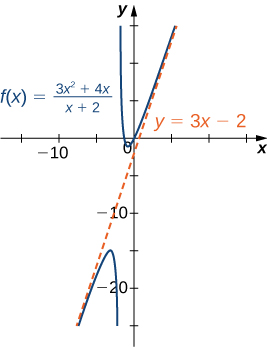Figure 17. The graph of the rational function $f(x)=(3{x}^{2}+4x)\text{/}(x+2)$ approaches the oblique asymptote $y=3x-2\text{as}x\to \pm \infty .$

Now let’s consider the end behavior for functions involving a radical.

### Determining End Behavior for a Function Involving a Radical

Find the limits as $x\to \infty$ and $x\to -\infty$ for $f(x)=\frac{3x-2}{\sqrt{4{x}^{2}+5}}$ and describe the end behavior of $f.$

#### Solution

Let’s use the same strategy as we did for rational functions: divide the numerator and denominator by a power of $x.$ To determine the appropriate power of $x,$ consider the expression $\sqrt{4{x}^{2}+5}$ in the denominator. Since

$\sqrt{4{x}^{2}+5}\approx \sqrt{4{x}^{2}}=2|x|$

for large values of $x$ in effect $x$ appears just to the first power in the denominator. Therefore, we divide the numerator and denominator by $|x|.$ Then, using the fact that $|x|=x$ for $x < 0,$ $|x|=-x$ for $x < 0,$ and $|x|=\sqrt{{x}^{2}}$ for all $x,$ we calculate the limits as follows:

$\begin{array}{ccc}\hfill \underset{x\to \infty }{\text{lim}}\frac{3x-2}{\sqrt{4{x}^{2}+5}}& =\hfill & \underset{x\to \infty }{\text{lim}}\frac{(1\text{/}|x|)(3x-2)}{(1\text{/}|x|)\sqrt{4{x}^{2}+5}}\hfill \\ & =\hfill & \underset{x\to \infty }{\text{lim}}\frac{(1\text{/}x)(3x-2)}{\sqrt{(1\text{/}{x}^{2})(4{x}^{2}+5)}}\hfill \\ & =\hfill & \underset{x\to \infty }{\text{lim}}\frac{3-2\text{/}x}{\sqrt{4+5\text{/}{x}^{2}}}=\frac{3}{\sqrt{4}}=\frac{3}{2}\hfill \\ \hfill \underset{x\to -\infty }{\text{lim}}\frac{3x-2}{\sqrt{4{x}^{2}+5}}& =\hfill & \underset{x\to -\infty }{\text{lim}}\frac{(1\text{/}|x|)(3x-2)}{(1\text{/}|x|)\sqrt{4{x}^{2}+5}}\hfill \\ & =\hfill & \underset{x\to -\infty }{\text{lim}}\frac{(-1\text{/}x)(3x-2)}{\sqrt{(1\text{/}{x}^{2})(4{x}^{2}+5)}}\hfill \\ & =\hfill & \underset{x\to -\infty }{\text{lim}}\frac{-3+2\text{/}x}{\sqrt{4+5\text{/}{x}^{2}}}=\frac{-3}{\sqrt{4}}=\frac{-3}{2}.\hfill \end{array}$

Therefore, $f(x)$ approaches the horizontal asymptote $y=\frac{3}{2}$ as $x\to \infty$ and the horizontal asymptote $y=-\frac{3}{2}$ as $x\to -\infty$ as shown in the following graph.

Evaluate $\underset{x\to \infty }{\text{lim}}\frac{\sqrt{3{x}^{2}+4}}{x+6}.$

#### Hint

Divide the numerator and denominator by $|x|.$

#### Solution

$\pm \sqrt{3}$

## Determining End Behavior for Transcendental Functions

The six basic trigonometric functions are periodic and do not approach a finite limit as $x\to \pm \infty .$ For example, $\sin x$ oscillates between $1\text{ and }-1$ ( (Figure) ). The tangent function $x$ has an infinite number of vertical asymptotes as $x\to \pm \infty ;$ therefore, it does not approach a finite limit nor does it approach $\pm \infty$ as $x\to \pm \infty$ as shown in (Figure) .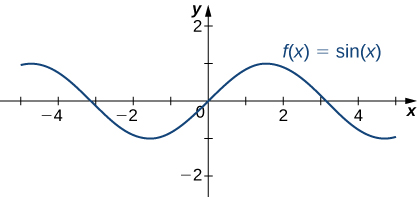Figure 19. The function $f(x)= \sin x$ oscillates between $1\text{ and }-1$ as $x\to \pm \infty$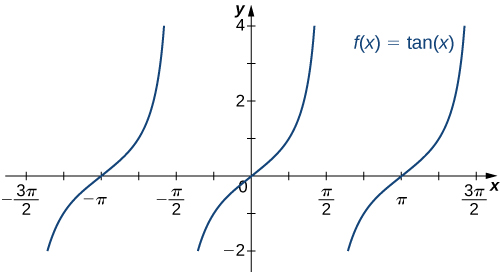Figure 20. The function $f(x)= \tan x$ does not approach a limit and does not approach $\pm \infty$ as $x\to \pm \infty$

Recall that for any base $b < 0,b\ne 1,$ the function $y={b}^{x}$ is an exponential function with domain $(-\infty ,\infty )$ and range $(0,\infty ).$ If $b < 1,y={b}^{x}$ is increasing over $`(-\infty ,\infty ).$ If $0 < b < 1,$ $y={b}^{x}$ is decreasing over $(-\infty ,\infty ).$ For the natural exponential function $f(x)={e}^{x},$ $e\approx 2.718 < 1.$ Therefore, $f(x)={e}^{x}$ is increasing on $`(-\infty ,\infty )$ and the range is $`(0,\infty ).$ The exponential function $f(x)={e}^{x}$ approaches $\infty$ as $x\to \infty$ and approaches 0 as $x\to -\infty$ as shown in (Figure) and (Figure) .

 $x$ -5 -2 0 2 5 ${e}^{x}$ 0.00674 0.135 1 7.389 148.413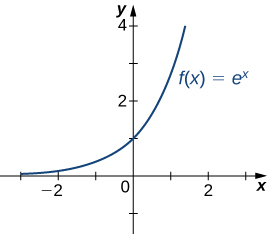Figure 21. The exponential function approaches zero as $x\to -\infty$ and approaches $\infty$ as $x\to \infty .$

Recall that the natural logarithm function $f(x)=\text{ln}(x)$ is the inverse of the natural exponential function $y={e}^{x}.$ Therefore, the domain of $f(x)=\text{ln}(x)$ is $(0,\infty )$ and the range is $(-\infty ,\infty ).$ The graph of $f(x)=\text{ln}(x)$ is the reflection of the graph of $y={e}^{x}$ about the line $y=x.$ Therefore, $\text{ln}(x)\to -\infty$ as $x\to {0}^{+}$ and $\text{ln}(x)\to \infty$ as $x\to \infty$ as shown in (Figure) and (Figure) .

 $x$ 0.01 0.1 1 10 100 $\text{ln}(x)$ -4.605 -2.303 0 2.303 4.605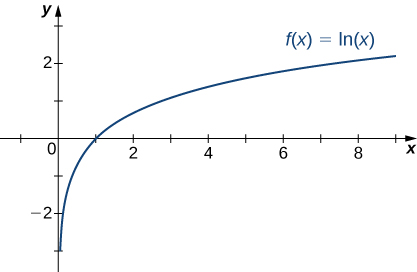Figure 22. The natural logarithm function approaches $\infty$ as $x\to \infty .$

### Determining End Behavior for a Transcendental Function

Find the limits as $x\to \infty$ and $x\to -\infty$ for $f(x)=\frac{(2+3{e}^{x})}{(7-5{e}^{x})}$ and describe the end behavior of $f.$

#### Solution

To find the limit as $x\to \infty ,$ divide the numerator and denominator by ${e}^{x}\text{:}$

$\begin{array}{cc}\hfill \underset{x\to \infty }{\text{lim}}f(x)& =\underset{x\to \infty }{\text{lim}}\frac{2+3{e}^{x}}{7-5{e}^{x}}\hfill \\ & =\underset{x\to \infty }{\text{lim}}\frac{(2\text{/}{e}^{x})+3}{(7\text{/}{e}^{x})-5}.\hfill \end{array}$

As shown in (Figure) , ${e}^{x}\to \infty$ as $x\to \infty .$ Therefore,

$\underset{x\to \infty }{\text{lim}}\frac{2}{{e}^{x}}=0=\underset{x\to \infty }{\text{lim}}\frac{7}{{e}^{x}}.$

We conclude that $\underset{x\to \infty }{\text{lim}}f(x)=-\frac{3}{5},$ and the graph of $f$ approaches the horizontal asymptote $y=-\frac{3}{5}$ as $x\to \infty .$ To find the limit as $x\to -\infty ,$ use the fact that ${e}^{x}\to 0$ as $x\to -\infty$ to conclude that $\underset{x\to \infty }{\text{lim}}f(x)=\frac{2}{7},$ and therefore the graph of approaches the horizontal asymptote $y=\frac{2}{7}$ as $x\to -\infty .$

Find the limits as $x\to \infty$ and $x\to -\infty$ for $f(x)=\frac{(3{e}^{x}-4)}{(5{e}^{x}+2)}.$

#### Hint

$\underset{x\to \infty }{\text{lim}}{e}^{x}=\infty$ and $\underset{x\to -\infty }{\text{lim}}{e}^{x}=0.$

#### Solution

$\underset{x\to \infty }{\text{lim}}f(x)=\frac{3}{5}$, $\underset{x\to -\infty }{\text{lim}}f(x)=-2$

### Key Concepts

• The limit of $f(x)$ is $L$ as $x\to \infty$ (or as $x\to -\infty )$ if the values $f(x)$ become arbitrarily close to $L$ as $x$ becomes sufficiently large.
• The limit of $f(x)$ is $\infty$ as $x\to \infty$ if $f(x)$ becomes arbitrarily large as $x$ becomes sufficiently large. The limit of $f(x)$ is $-\infty$ as $x\to \infty$ if $f(x) < 0$ and $|f(x)|$ becomes arbitrarily large as $x$ becomes sufficiently large. We can define the limit of $f(x)$ as $x$ approaches $-\infty$ similarly.

For the following exercises, examine the graphs. Identify where the vertical asymptotes are located.

1.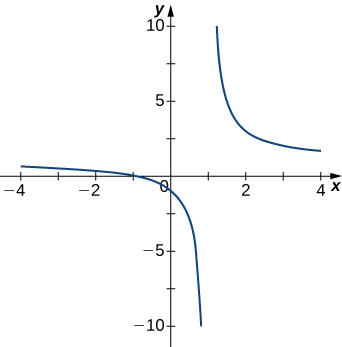#### Solution

$x=1$

2.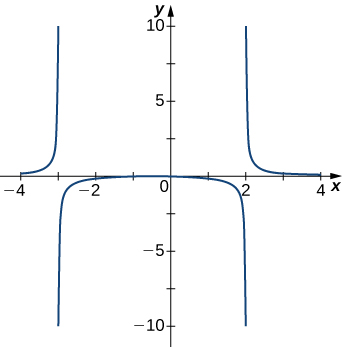3.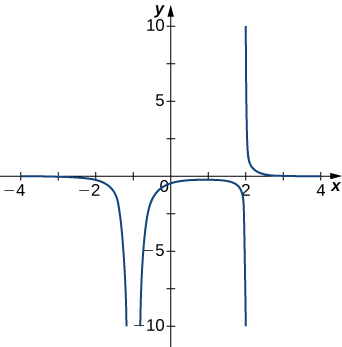#### Solution

$x=-1,x=2$

4.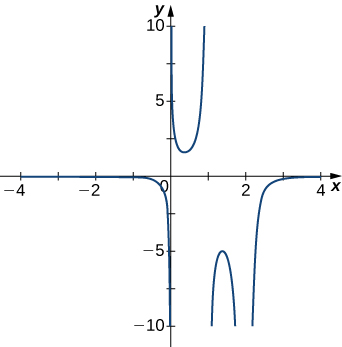5.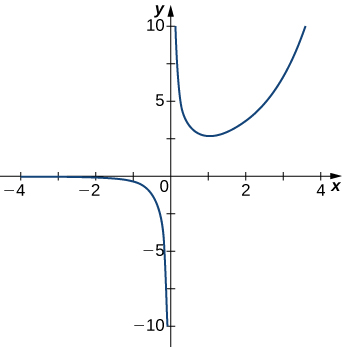#### Solution

$x=0$

For the following functions $f(x),$ determine whether there is an asymptote at $x=a.$ Justify your answer without graphing on a calculator.

6. $f(x)=\frac{x+1}{{x}^{2}+5x+4},a=-1$

7. $f(x)=\frac{x}{x-2},a=2$

#### Solution

Yes, there is a vertical asymptote

8. $f(x)={(x+2)}^{3\text{/}2},a=-2$

9. $f(x)={(x-1)}^{-1\text{/}3},a=1$

#### Solution

Yes, there is vertical asymptote

10. $f(x)=1+{x}^{-2\text{/}5},a=1$

For the following exercises, evaluate the limit.

11. $\underset{x\to \infty }{\text{lim}}\frac{1}{3x+6}$

#### Solution

0

12. $\underset{x\to \infty }{\text{lim}}\frac{2x-5}{4x}$

13. $\underset{x\to \infty }{\text{lim}}\frac{{x}^{2}-2x+5}{x+2}$

#### Solution

$\infty$

14. $\underset{x\to -\infty }{\text{lim}}\frac{3{x}^{3}-2x}{{x}^{2}+2x+8}$

15. $\underset{x\to -\infty }{\text{lim}}\frac{{x}^{4}-4{x}^{3}+1}{2-2{x}^{2}-7{x}^{4}}$

#### Solution

$-\frac{1}{7}$

16. $\underset{x\to \infty }{\text{lim}}\frac{3x}{\sqrt{{x}^{2}+1}}$

17. $\underset{x\to -\infty }{\text{lim}}\frac{\sqrt{4{x}^{2}-1}}{x+2}$

#### Solution

-2

18. $\underset{x\to \infty }{\text{lim}}\frac{4x}{\sqrt{{x}^{2}-1}}$

19. $\underset{x\to -\infty }{\text{lim}}\frac{4x}{\sqrt{{x}^{2}-1}}$

#### Solution

-4

20. $\underset{x\to \infty }{\text{lim}}\frac{2\sqrt{x}}{x-\sqrt{x}+1}$

21. $\underset{x\to \infty }{\text{lim}}\frac{3-x}{\sqrt{x-2}}$

#### Solution

$-\infty$

22. $\underset{x\to \infty }{\text{lim}}(\sqrt{x^2+5x-1}-x)$

23. $\underset{x\to -\infty }{\text{lim}}(\sqrt{x^2 +4x}+x)$

#### Solution

$-2$

24. $\underset{x\to \infty }{\text{lim}}(\sqrt{4x^2+3x}-2x)$

For the following exercises, find the horizontal and vertical asymptotes.

25. $f(x)=x-\frac{9}{x}$

#### Solution

Horizontal: none, vertical: $x=0$

26. $f(x)=\frac{1}{1-{x}^{2}}$

27. $f(x)=\frac{{x}^{3}}{4-{x}^{2}}$

#### Solution

Horizontal: none, vertical: $x=\pm 2$

28. $f(x)=\frac{{x}^{2}+3}{{x}^{2}+1}$

29. $f(x)= \sin (x) \sin (2x)$

#### Solution

Horizontal: none, vertical: none

30. $f(x)= \cos x+ \cos (3x)+ \cos (5x)$

31. $f(x)=\frac{x \sin (x)}{{x}^{2}-1}$

#### Solution

Horizontal: $y=0,$ vertical: $x=\pm 1$

32. $f(x)=\frac{x}{ \sin (x)}$

33. $f(x)=\frac{1}{{x}^{3}+{x}^{2}}$

#### Solution

Horizontal: $y=0,$ vertical: $x=0$ and $x=-1$

34. $f(x)=\frac{1}{x-1}-2x$

35. $f(x)=\frac{{x}^{3}+1}{{x}^{3}-1}$

#### Solution

Horizontal: $y=1,$ vertical: $x=1$

36. $f(x)=\frac{ \sin x+ \cos x}{ \sin x- \cos x}$

37. $f(x)=x- \sin x$

#### Solution

Horizontal: none, vertical: none

38. $f(x)=\frac{1}{x}-\sqrt{x}$

39. $f(x) = \frac{x}{\sqrt{x^3+1}}$

#### Solution

Horizontal: $y=1$, vertical: $x=-1$

40. $f(x)= \frac{x-2}{\sqrt{3x^2+2x-1}}$

For the following exercises, construct a function $f(x)$ that has the given asymptotes.

41. $x=1$ and $y=2$

#### Solution

Answers will vary, for example: $y=\frac{2x}{x-1}$

42. $x=1$ and $y=0$

43. $y=4$ and $x=-1$

#### Solution

Answers will vary, for example: $y=\frac{4x}{x+1}$

44. $x=0$

For the following exercises, graph the function on a graphing calculator on the window $x=\left[-5,5\right]$ and estimate the horizontal asymptote or limit. Then, calculate the actual horizontal asymptote or limit.

45. [T] $f(x)=\frac{1}{x+10}$

#### Solution

$y=0$

46. [T] $f(x)=\frac{x+1}{{x}^{2}+7x+6}$

47. [T] $\underset{x\to -\infty }{\text{lim}}{x}^{2}+10x+25$

#### Solution

$\infty$

48. [T] $\underset{x\to -\infty }{\text{lim}}\frac{x+2}{{x}^{2}+7x+6}$

49. [T] $\underset{x\to \infty }{\text{lim}}\frac{3x+2}{x+5}$

#### Solution

$y=3$

50. True or false: Every ratio of polynomials has vertical asymptotes.

## Glossary

end behavior
the behavior of a function as $x\to \infty$ and $x\to -\infty$
horizontal asymptote
if $\underset{x\to \infty }{\text{lim}}f(x)=L$ or $\underset{x\to -\infty }{\text{lim}}f(x)=L,$ then $y=L$ is a horizontal asymptote of $f$
infinite limit at infinity
a function that becomes arbitrarily large as $x$ becomes large
limit at infinity
the limiting value, if it exists, of a function as $x\to \infty$ or $x\to -\infty$
oblique asymptote
the line $y=mx+b$ if $f(x)$ approaches it as $x\to \infty$ or $x\to -\infty$